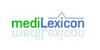logitThe logarithm of the ratio of frequencies of two different categorical and mutually exclusive outcomes such as healthy and sick. ... (05 Mar 2000) ...
Found on http://www.encyclo.co.uk/local/20973

logitType: Term Pronunciation: lō′git Definitions: 1. The logarithm of the ratio of frequencies of two different categoric and mutually exclusive outcomes such as healthy and sick.
Found on http://www.medilexicon.com/medicaldictionary.php?t=51274

LogitThe logit (t {respell|LOH|jit}) function is the inverse of the sigmoidal `logistic` function or logistic transform used in mathematics, especially in statistics. When the function`s parameter represents a probability {mvar|p}, the logit function gives the log-odds, or the logarithm of the odds {mvar|p}/(1-{mvar|p}). ==History== Log odds was use......
Found on http://en.wikipedia.org/wiki/Logit
No exact match found